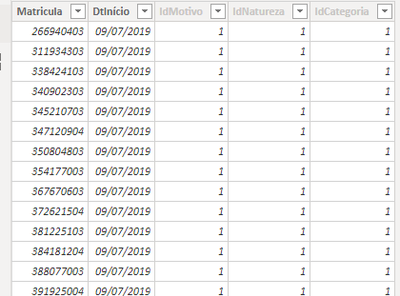cancel
Showing results for
Did you mean:New Member

## Counting based on another field

Hi!

I would like to create a measure that is able to count the number of Matriculas in which:

1. the IdMotivo is equal to 1 and

2. there is a IdNatureza equals to 4 whatever the date it occurs.

The measure should output in a given month, created by filter context, the number of IdMotivo = 1 in that month for which there was a IdNatureza = 4 no matter the date this last one occured.1 ACCEPTED SOLUTIONSuper User

@douglas-verdum So generally you would have a relationship between your date table and that table based on date and you would use Month or something like that from your date table in the axis of your visual. Also, if the = 4 part can be in any month, you would need a slight variation:

``````Measure =
VAR __Table = DISTINCT(SELECTCOLUMNS(FILTER(ALL('Table'), [IdNatureza] = 4), "__Matricula", [Matricula]))
VAR __Table1 = DISTINCT(SELECTCOLUMNS(FILTER('Table', [IdMotivo] = 1), "__Matricula", [Matricula]))
RETURN
COUNTROWS(INTERSECT(__Table1, __Table))``````

Become an expert!: Enterprise DNA
External Tools: MSHGQM
Latest book!:
Mastering Power BI 2nd EditionDAX is easy, CALCULATE makes DAX hard...
4 REPLIES 4Super User

@douglas-verdum Maybe something like:

``````Measure =
VAR __Table = DISTINCT(SELECTCOLUMNS(FILTER('Table', [IdNatureza] = 4), "__Matricula", [Matricula]))
VAR __Table1 = DISTINCT(SELECTCOLUMNS(FILTER('Table', [IdMotivo] = 1), "__Matricula", [Matricula]))
RETURN
COUNTROWS(INTERSECT(__Table1, __Table))``````

Become an expert!: Enterprise DNA
External Tools: MSHGQM
Latest book!:
Mastering Power BI 2nd EditionDAX is easy, CALCULATE makes DAX hard...New Member

great @Greg_Deckler

but this gives the aggregate value

could you come up with something that splits this number along the date filter context in a view?

there is a dimCalendar related to this fact table

thanks!Super User

@douglas-verdum So generally you would have a relationship between your date table and that table based on date and you would use Month or something like that from your date table in the axis of your visual. Also, if the = 4 part can be in any month, you would need a slight variation:

``````Measure =
VAR __Table = DISTINCT(SELECTCOLUMNS(FILTER(ALL('Table'), [IdNatureza] = 4), "__Matricula", [Matricula]))
VAR __Table1 = DISTINCT(SELECTCOLUMNS(FILTER('Table', [IdMotivo] = 1), "__Matricula", [Matricula]))
RETURN
COUNTROWS(INTERSECT(__Table1, __Table))``````

Become an expert!: Enterprise DNA
External Tools: MSHGQM
Latest book!:
Mastering Power BI 2nd EditionDAX is easy, CALCULATE makes DAX hard...New Member

perfect, @Greg_Deckler

it worked perfectly

thanks a lot